# Angles

Print
Rate 0 stars
Common Core
• Lesson size:
Message preview:
Someone you know has shared lesson with you:

To play this lesson, click on the link below:

https://www.turtlediary.com/lesson/angles.html

Hope you have a good experience with this site and recommend to your friends too.

Login to rate activities and track progress.
Login to rate activities and track progress.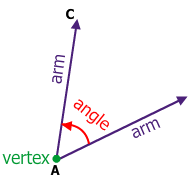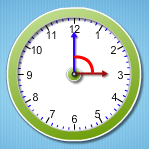• Angles are formed when two rays meet. If there are two straight lines such that they have a common end point, then the amount of curl between these two lines is known as 'angle'.
• There are angles in most shapes and in many of the objects you see and use on a daily basis.
• Angles are measured in degrees.
• There are four types of angles:
• Acute angle
• Obtuse angle
• Right angle
• Straight angle

## Right angle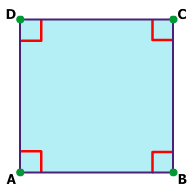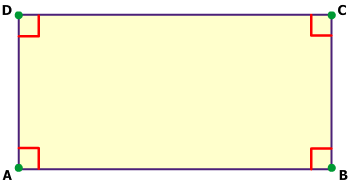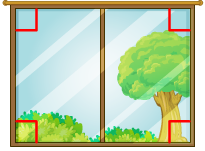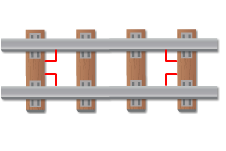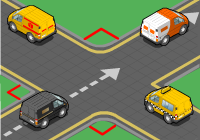• When two straight lines are perpendicular to each other or make 90° with each other, then they are said to be at right angles.
• A right angle is exactly 90°.
• Right angles are often shown with a box in the corner.
• Squares and rectangles are formed using right angles.
• Some real life examples of Right angles are :-

## Acute angle

When two straight lines make an angle with each other such that it is greater than 0° and less than 90°, then these lines are said to be making an acute angle with each other.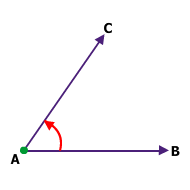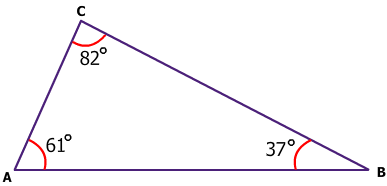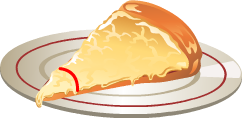• Acute angles are less than 90°.
• Some triangles have acute angles.
• Here are few examples: The slice of pizza that you eat for lunch is an acute angle.
• You get a new box of pencils for school. After you sharpen all of the pencils you notice that the tips are acute angles.

## Obtuse angle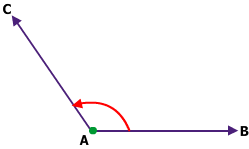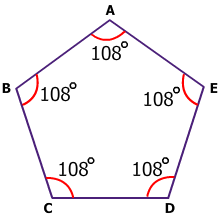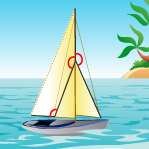• When two straight lines make an angle with each other such that it is greater than 90° and less than 180°, then these lines are said to be making an obtuse angle with each other.
• Obtuse angles are more than 90° but less than 180°.
• Many shapes such as pentagons, octagons and triangles have obtuse angles.
• Lets see some examples of obtuse angles.

## Straight angle• When two line segments point exactly in the opposite direction and form a single straight line, then the angle they form is 180° or straight angle.

## Angles

• Angles are formed when two rays meet.
• If there are two straight rays such that they have a common end point, then the amount of curl between these two lines is known as 'angle'.
• There are four types of angles:
• Acute angle
• Obtuse angle
• Right angle
• Straight angle
• When two straight lines are perpendicular to each other or make 90° with each other, then they are said to be at right angles.
• When two straight lines make an angle with each other such that it is greater than 0° and less than 90°, then these lines are said to be making an acute angle with each other.
• When two straight lines make an angle with each other such that it is greater than 90° and less than 180°, then these lines are said to be making an obtuse angle with each other.
• When two line segments point exactly in the opposite direction and form a single straight line, then the angle they form is 180° or straight angle.
Become premium member to get unlimited access.